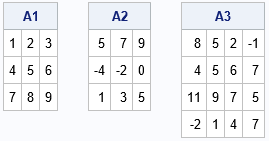Look at the following matrices. Do you notice anything that these matrices have in common?If you noticed that the rows of each matrix are arithmetic progressions, good for you. For each row, there is a constant difference (also called the "increment") between adjacent elements. For these examples:

• In the first matrix, each row is an arithmetic progression with a difference of 1. That is, the value of the (j+1)th column is one more than the value of the j_th column.
• In the second matrix, each row is an arithmetic progression with a difference of 2. That is, the value of the (j+1)th column is two more than the value of the j_th column.
• In the third matrix, each row is an arithmetic progression, but the increments for the rows are not identical. The increment for the first row is -3. The increment for the second row is +1. The increments for the remaining rows are -2 and +3, respectively.

However, there is another characteristic that these matrices have in common: they are all singular matrices. It turns out this is not a coincidence. An n x n matrix (n>2) is singular if each row is an arithmetic progression.

### Why are these matrices singular?

Recall that a matrix will be singular if one of the columns can be written as a linear combination of other columns. For these three matrices, you can use mental arithmetic to verify that the third column is a linear combination of the first two columns. Specifically, you can verify that the third column can be written as
X3 = 2 X2 – X1
where X1, X2, X3, ... are the columns of a matrix. Thus, these matrices are singular.

It turns out that this relationship holds in general for any matrix whose rows are arithmetic progressions. Suppose the elements of the i_th row satisfy the progression with increment di so that the elements of the i_th row satisfy X[i, j+1] = X[i, j] + d[i]  for j=1,2,...,n. Let d be the vector of differences. Then
X2 = X1 + d
which you can solve for d to obtain
d = X2 – X1.
By substitution,
X3 = X2 + d = X2 + (X2 – X1), or
X3 = 2*X2 – X1.
Therefore, the third column is always a linear combination of the first two columns, and the coefficients are independent of the differences in the arithmetic progressions of the rows. It doesn't matter what increments are used in the arithmetic progressions of the rows.

In fact, even more is true. You can use similar arguments to show that the j_th column can be written as a linear combination of the first two columns for any j > 2. Thus, regardless of the matrix size, matrices whose rows are arithmetic progressions are rank-2 matrices: they have only two linearly independent columns.